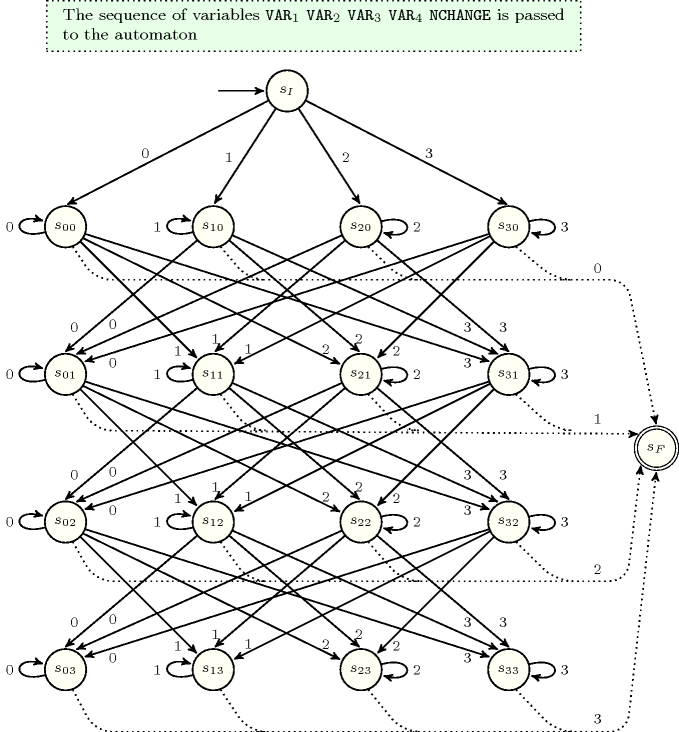## 5.61. change

Origin

CHIP

Constraint

$\mathrm{𝚌𝚑𝚊𝚗𝚐𝚎}\left(\mathrm{𝙽𝙲𝙷𝙰𝙽𝙶𝙴},\mathrm{𝚅𝙰𝚁𝙸𝙰𝙱𝙻𝙴𝚂},\mathrm{𝙲𝚃𝚁}\right)$

Synonyms

$\mathrm{𝚗𝚋𝚌𝚑𝚊𝚗𝚐𝚎𝚜}$, $\mathrm{𝚜𝚒𝚖𝚒𝚕𝚊𝚛𝚒𝚝𝚢}$.

Arguments
 $\mathrm{𝙽𝙲𝙷𝙰𝙽𝙶𝙴}$ $\mathrm{𝚍𝚟𝚊𝚛}$ $\mathrm{𝚅𝙰𝚁𝙸𝙰𝙱𝙻𝙴𝚂}$ $\mathrm{𝚌𝚘𝚕𝚕𝚎𝚌𝚝𝚒𝚘𝚗}\left(\mathrm{𝚟𝚊𝚛}-\mathrm{𝚍𝚟𝚊𝚛}\right)$ $\mathrm{𝙲𝚃𝚁}$ $\mathrm{𝚊𝚝𝚘𝚖}$
Restrictions
 $\mathrm{𝙽𝙲𝙷𝙰𝙽𝙶𝙴}\ge 0$ $\mathrm{𝙽𝙲𝙷𝙰𝙽𝙶𝙴}<|\mathrm{𝚅𝙰𝚁𝙸𝙰𝙱𝙻𝙴𝚂}|$ $\mathrm{𝚛𝚎𝚚𝚞𝚒𝚛𝚎𝚍}$$\left(\mathrm{𝚅𝙰𝚁𝙸𝙰𝙱𝙻𝙴𝚂},\mathrm{𝚟𝚊𝚛}\right)$ $\mathrm{𝙲𝚃𝚁}\in \left[=,\ne ,<,\ge ,>,\le \right]$
Purpose

$\mathrm{𝙽𝙲𝙷𝙰𝙽𝙶𝙴}$ is the number of times that constraint $\mathrm{𝙲𝚃𝚁}$ holds on consecutive variables of the collection $\mathrm{𝚅𝙰𝚁𝙸𝙰𝙱𝙻𝙴𝚂}$.

Example
 $\left(3,〈4,4,3,4,1〉,\ne \right)$ $\left(1,〈1,2,4,3,7〉,>\right)$

In the first example the changes are located between values 4 and 3, 3 and 4, 4 and 1. Consequently, the corresponding $\mathrm{𝚌𝚑𝚊𝚗𝚐𝚎}$ constraint holds since its first argument $\mathrm{𝙽𝙲𝙷𝙰𝙽𝙶𝙴}$ is fixed to value 3.

In the second example the unique change occurs between values 4 and 3. Consequently, the corresponding $\mathrm{𝚌𝚑𝚊𝚗𝚐𝚎}$ constraint holds since its first argument $\mathrm{𝙽𝙲𝙷𝙰𝙽𝙶𝙴}$ is fixed to 1.

All solutions

Figure 5.61.1 gives all solutions to the following non ground instance of the $\mathrm{𝚌𝚑𝚊𝚗𝚐𝚎}$ constraint: $\mathrm{𝙽𝙲𝙷𝙰𝙽𝙶𝙴}\in \left[0,1\right]$, ${V}_{1}\in \left[2,3\right]$, ${V}_{2}\in \left[1,2\right]$, ${V}_{3}\in \left[4,5\right]$, ${V}_{4}\in \left[2,4\right]$, $\mathrm{𝚌𝚑𝚊𝚗𝚐𝚎}$$\left(\mathrm{𝙽𝙲𝙷𝙰𝙽𝙶𝙴},〈{V}_{1},{V}_{2},{V}_{3},{V}_{4}〉,>\right)$.

##### Figure 5.61.1. All solutions corresponding to the non ground example of the $\mathrm{𝚌𝚑𝚊𝚗𝚐𝚎}$ constraint (with $\mathrm{𝙲𝚃𝚁}$ set to $>$) of the All solutions slot; each change is shown by a color change between two consecutive values.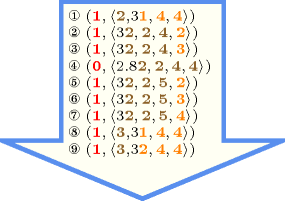Typical
 $\mathrm{𝙽𝙲𝙷𝙰𝙽𝙶𝙴}>0$ $|\mathrm{𝚅𝙰𝚁𝙸𝙰𝙱𝙻𝙴𝚂}|>1$ $\mathrm{𝚛𝚊𝚗𝚐𝚎}$$\left(\mathrm{𝚅𝙰𝚁𝙸𝙰𝙱𝙻𝙴𝚂}.\mathrm{𝚟𝚊𝚛}\right)>1$ $\mathrm{𝙲𝚃𝚁}\in \left[\ne \right]$
Symmetry

One and the same constant can be added to the $\mathrm{𝚟𝚊𝚛}$ attribute of all items of $\mathrm{𝚅𝙰𝚁𝙸𝙰𝙱𝙻𝙴𝚂}$.

Arg. properties
• Functional dependency: $\mathrm{𝙽𝙲𝙷𝙰𝙽𝙶𝙴}$ determined by $\mathrm{𝚅𝙰𝚁𝙸𝙰𝙱𝙻𝙴𝚂}$ and $\mathrm{𝙲𝚃𝚁}$.

• Contractible wrt. $\mathrm{𝚅𝙰𝚁𝙸𝙰𝙱𝙻𝙴𝚂}$ when $\mathrm{𝙲𝚃𝚁}\in \left[\ne ,<,\ge ,>,\le \right]$ and $\mathrm{𝙽𝙲𝙷𝙰𝙽𝙶𝙴}=0$.

• Contractible wrt. $\mathrm{𝚅𝙰𝚁𝙸𝙰𝙱𝙻𝙴𝚂}$ when $\mathrm{𝙲𝚃𝚁}\in \left[=,<,\ge ,>,\le \right]$ and $\mathrm{𝙽𝙲𝙷𝙰𝙽𝙶𝙴}=|\mathrm{𝚅𝙰𝚁𝙸𝙰𝙱𝙻𝙴𝚂}|-1$.

Usage

This constraint can be used in the context of timetabling problems in order to put an upper limit on the number of changes of job types during a given period.

Remark

A similar constraint appears in [PachetRoy99] under the name of $\mathrm{𝚜𝚒𝚖𝚒𝚕𝚊𝚛𝚒𝚝𝚢}$ constraint. The difference consists of replacing the arithmetic constraint $\mathrm{𝙲𝚃𝚁}$ by a binary constraint. When $\mathrm{𝙲𝚃𝚁}$ is equal to $\ne$ this constraint is called $\mathrm{𝚗𝚋𝚌𝚑𝚊𝚗𝚐𝚎𝚜}$ in [Platon03].

Algorithm

A first incomplete algorithm is described in [BeldiceanuCarlsson01]. The sketch of a filtering algorithm for the conjunction of the $\mathrm{𝚌𝚑𝚊𝚗𝚐𝚎}$ and the $\mathrm{𝚜𝚝𝚛𝚎𝚝𝚌𝚑}$ constraints based on dynamic programming achieving arc-consistency is mentioned by L. Hellsten in [Hellsten04].

Reformulation

The $\mathrm{𝚌𝚑𝚊𝚗𝚐𝚎}$ constraint can be reformulated with the $\mathrm{𝚜𝚎𝚚}_\mathrm{𝚋𝚒𝚗}$ constraint [PetitBeldiceanuLorca11] that we now introduce. Given $𝙽$ a domain variable, $𝚇$ a sequence of domain variables, and $𝙲$ and $𝙱$ two binary constraints, $\mathrm{𝚜𝚎𝚚}_\mathrm{𝚋𝚒𝚗}$$\left(𝙽,𝚇,𝙲,𝙱\right)$ holds if (1) $𝙽$ is equal to the number of $𝙲$-stretches in the sequence $𝚇$, and (2) $𝙱$ holds on any pair of consecutive variables in $𝚇$. A $𝙲$-stretch is a generalisation of the notion of stretch introduced by G. Pesant [Pesant01], where the equality constraint is made explicit by replacing it by a binary constraint $𝙲$, i.e., a $𝙲$-stretch is a maximal length subsequence of $𝚇$ for which the binary constraint $𝙲$ is satisfied on consecutive variables. $\mathrm{𝚌𝚑𝚊𝚗𝚐𝚎}$$\left(\mathrm{𝙽𝙲𝙷𝙰𝙽𝙶𝙴},\mathrm{𝚅𝙰𝚁𝙸𝙰𝙱𝙻𝙴𝚂},\mathrm{𝙲𝚃𝚁}\right)$ can be reformulated as $𝙽=𝙽\mathtt{1}-1\wedge$$\mathrm{𝚜𝚎𝚚}_\mathrm{𝚋𝚒𝚗}$$\left(𝙽\mathtt{1},𝚇,¬\mathrm{𝙲𝚃𝚁},\mathrm{true}\right)$, where $\mathrm{true}$ is the universal constraint.

Used in

common keyword: $\mathrm{𝚌𝚑𝚊𝚗𝚐𝚎}_\mathrm{𝚙𝚊𝚛𝚝𝚒𝚝𝚒𝚘𝚗}$, $\mathrm{𝚌𝚒𝚛𝚌𝚞𝚕𝚊𝚛}_\mathrm{𝚌𝚑𝚊𝚗𝚐𝚎}$ (number of changes in a sequence of $\mathrm{𝚟𝚊𝚛𝚒𝚊𝚋𝚕𝚎𝚜}$ with respect to a $\mathrm{𝚋𝚒𝚗𝚊𝚛𝚢}\mathrm{𝚌𝚘𝚗𝚜𝚝𝚛𝚊𝚒𝚗𝚝}$), $\mathrm{𝚌𝚢𝚌𝚕𝚒𝚌}_\mathrm{𝚌𝚑𝚊𝚗𝚐𝚎}$, $\mathrm{𝚌𝚢𝚌𝚕𝚒𝚌}_\mathrm{𝚌𝚑𝚊𝚗𝚐𝚎}_\mathrm{𝚓𝚘𝚔𝚎𝚛}$ (number of changes), $\mathrm{𝚜𝚖𝚘𝚘𝚝𝚑}$ (number of changes in a sequence of $\mathrm{𝚟𝚊𝚛𝚒𝚊𝚋𝚕𝚎𝚜}$ with respect to a $\mathrm{𝚋𝚒𝚗𝚊𝚛𝚢}\mathrm{𝚌𝚘𝚗𝚜𝚝𝚛𝚊𝚒𝚗𝚝}$).

generalisation: $\mathrm{𝚌𝚑𝚊𝚗𝚐𝚎}_\mathrm{𝚙𝚊𝚒𝚛}$ ($\mathrm{𝚟𝚊𝚛𝚒𝚊𝚋𝚕𝚎}$ replaced by $\mathrm{𝚙𝚊𝚒𝚛}$ of $\mathrm{𝚟𝚊𝚛𝚒𝚊𝚋𝚕𝚎𝚜}$), $\mathrm{𝚌𝚑𝚊𝚗𝚐𝚎}_\mathrm{𝚟𝚎𝚌𝚝𝚘𝚛𝚜}$ ($\mathrm{𝚟𝚊𝚛𝚒𝚊𝚋𝚕𝚎}$ replaced by vector).

Keywords
Arc input(s)

$\mathrm{𝚅𝙰𝚁𝙸𝙰𝙱𝙻𝙴𝚂}$

Arc generator
$\mathrm{𝑃𝐴𝑇𝐻}$$↦\mathrm{𝚌𝚘𝚕𝚕𝚎𝚌𝚝𝚒𝚘𝚗}\left(\mathrm{𝚟𝚊𝚛𝚒𝚊𝚋𝚕𝚎𝚜}\mathtt{1},\mathrm{𝚟𝚊𝚛𝚒𝚊𝚋𝚕𝚎𝚜}\mathtt{2}\right)$

Arc arity
Arc constraint(s)
$\mathrm{𝚟𝚊𝚛𝚒𝚊𝚋𝚕𝚎𝚜}\mathtt{1}.\mathrm{𝚟𝚊𝚛}\mathrm{𝙲𝚃𝚁}\mathrm{𝚟𝚊𝚛𝚒𝚊𝚋𝚕𝚎𝚜}\mathtt{2}.\mathrm{𝚟𝚊𝚛}$
Graph property(ies)
$\mathrm{𝐍𝐀𝐑𝐂}$$=\mathrm{𝙽𝙲𝙷𝙰𝙽𝙶𝙴}$

Graph class
 $•$$\mathrm{𝙰𝙲𝚈𝙲𝙻𝙸𝙲}$ $•$$\mathrm{𝙱𝙸𝙿𝙰𝚁𝚃𝙸𝚃𝙴}$ $•$$\mathrm{𝙽𝙾}_\mathrm{𝙻𝙾𝙾𝙿}$

Graph model

Since we are only interested by the constraints linking two consecutive items of the collection $\mathrm{𝚅𝙰𝚁𝙸𝙰𝙱𝙻𝙴𝚂}$ we use $\mathrm{𝑃𝐴𝑇𝐻}$ to generate the arcs of the initial graph.

Parts (A) and (B) of Figure 5.61.2 respectively show the initial and final graph of the first example of the Example slot. Since we use the $\mathrm{𝐍𝐀𝐑𝐂}$ graph property, the arcs of the final graph are stressed in bold.

##### Figure 5.61.2. Initial and final graph of the $\mathrm{𝚌𝚑𝚊𝚗𝚐𝚎}$ constraint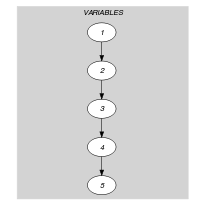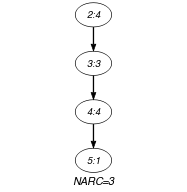(a) (b)
Automaton

Figure 5.61.3 depicts a first automaton that only accepts all the solutions to the $\mathrm{𝚌𝚑𝚊𝚗𝚐𝚎}$ constraint. This automaton uses a counter in order to record the number of satisfied constraints of the form ${\mathrm{𝚅𝙰𝚁}}_{i}\mathrm{𝙲𝚃𝚁}{\mathrm{𝚅𝙰𝚁}}_{i+1}$ already encountered. To each pair of consecutive variables $\left({\mathrm{𝚅𝙰𝚁}}_{i},{\mathrm{𝚅𝙰𝚁}}_{i+1}\right)$ of the collection $\mathrm{𝚅𝙰𝚁𝙸𝙰𝙱𝙻𝙴𝚂}$ corresponds a 0-1 signature variable ${S}_{i}$. The following signature constraint links ${\mathrm{𝚅𝙰𝚁}}_{i}$, ${\mathrm{𝚅𝙰𝚁}}_{i+1}$ and ${S}_{i}$: ${\mathrm{𝚅𝙰𝚁}}_{i}\mathrm{𝙲𝚃𝚁}{\mathrm{𝚅𝙰𝚁}}_{i+1}⇔{S}_{i}$.

##### Figure 5.61.3. Automaton (with counter) of the $\mathrm{𝚌𝚑𝚊𝚗𝚐𝚎}$ constraint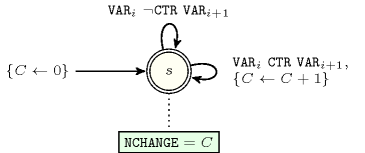##### Figure 5.61.4. Hypergraph of the reformulation corresponding to the automaton (with counter) of the $\mathrm{𝚌𝚑𝚊𝚗𝚐𝚎}$ constraint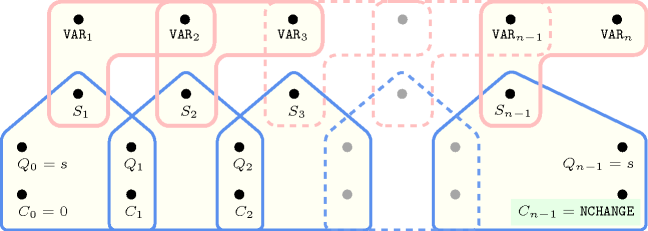Since the reformulation associated with the previous automaton is not Berge-acyclic, we now describe a second counter free automaton that also only accepts all the solutions to the $\mathrm{𝚌𝚑𝚊𝚗𝚐𝚎}$ constraint. Without loss of generality, assume that the collection of variables $\mathrm{𝚅𝙰𝚁𝙸𝙰𝙱𝙻𝙴𝚂}$ contains at least two variables (i.e., $|\mathrm{𝚅𝙰𝚁𝙸𝙰𝙱𝙻𝙴𝚂}|\ge 2$). Let $n$ and $𝒟$ respectively denote the number of variables of the collection $\mathrm{𝚅𝙰𝚁𝙸𝙰𝙱𝙻𝙴𝚂}$, and the union of the domains of the variables of $\mathrm{𝚅𝙰𝚁𝙸𝙰𝙱𝙻𝙴𝚂}$. Clearly, the maximum number of changes (i.e., the number of times the constraint ${\mathrm{𝚅𝙰𝚁}}_{i}\mathrm{𝙲𝚃𝚁}{\mathrm{𝚅𝙰𝚁}}_{i+1}$ $\left(1\le i holds) cannot exceed the quantity $m=min\left(n-1,\overline{\mathrm{𝙽𝙲𝙷𝙰𝙽𝙶𝙴}}\right)$. The $\left(m+1\right)·|𝒟|+2$ states of the automaton that only accepts all the solutions to the $\mathrm{𝚌𝚑𝚊𝚗𝚐𝚎}$ constraint are defined in the following way:

• We have an initial state labelled by ${s}_{I}$.

• We have $m·|𝒟|$ intermediate states labelled by ${s}_{ij}$ $\left(i\in 𝒟,j\in \left[0,m\right]\right)$. The first subscript $i$ of state ${s}_{ij}$ corresponds to the value currently encountered. The second subscript $j$ denotes the number of already encountered satisfied constraints of the form ${\mathrm{𝚅𝙰𝚁}}_{i}\mathrm{𝙲𝚃𝚁}{\mathrm{𝚅𝙰𝚁}}_{i+1}$ from the initial state ${s}_{I}$ to the state ${s}_{ij}$.

• We have an accepting state labelled by ${s}_{F}$.

Four classes of transitions are respectively defined in the following way:

1. There is a transition, labelled by $i$ from the initial state ${s}_{I}$ to the state ${s}_{i0}$, $\left(i\in 𝒟\right)$.

2. There is a transition, labelled by $j$, from every state ${s}_{ij}$, $\left(i\in 𝒟,j\in \left[0,m\right]\right)$, to the accepting state ${s}_{F}$.

3. $\forall i\in 𝒟,\forall j\in \left[0,m\right],\forall k\in 𝒟\cap \left\{k|i¬\mathrm{𝙲𝚃𝚁}k\right\}$ there is a transition labelled by $k$ from ${s}_{ij}$ to ${s}_{kj}$ (i.e., the counter $j$ does not change for values $k$ such that constraint $i\mathrm{𝙲𝚃𝚁}k$ does not hold).

4. $\forall i\in 𝒟,\forall j\in \left[0,m-1\right],\forall k\in 𝒟\setminus \left\{k|i¬\mathrm{𝙲𝚃𝚁}k\right\}$ there is a transition labelled by $k$ from ${s}_{ij}$ to ${s}_{kj+1}$ (i.e., the counter $j$ is incremented by $+1$ for values $k$ such that constraint $i\mathrm{𝙲𝚃𝚁}k$ holds).

We have $|𝒟|$ transitions of type 1, $|𝒟|·\left(m+1\right)$ transitions of type 2, and at least ${|𝒟|}^{2}·m$ transitions of types 3 and 4. Since the maximum value of $m$ is equal to $n-1$, in the worst case we have at least ${|𝒟|}^{2}·\left(n-1\right)$ transitions. This leads to a worst case time complexity of $O\left(|𝒟{|}^{2}·{n}^{2}\right)$ if we use Pesant's algorithm for filtering the $\mathrm{𝚛𝚎𝚐𝚞𝚕𝚊𝚛}$ constraint [Pesant04].

Figure 5.61.5 depicts the corresponding counter free non deterministic automaton associated with the $\mathrm{𝚌𝚑𝚊𝚗𝚐𝚎}$ constraint under the hypothesis that (1) all variables of $\mathrm{𝚅𝙰𝚁𝙸𝙰𝙱𝙻𝙴𝚂}$ are assigned a value in $\left\{0,1,2,3\right\}$, (2) $|\mathrm{𝚅𝙰𝚁𝙸𝙰𝙱𝙻𝙴𝚂}|$ is equal to 4, and (3) $\mathrm{𝙲𝚃𝚁}$ is equal to $\ne$.

##### Figure 5.61.5. Counter free non deterministic automaton of the $\mathrm{𝚌𝚑𝚊𝚗𝚐𝚎}$$\left(\mathrm{𝙽𝙲𝙷𝙰𝙽𝙶𝙴},〈{\mathrm{𝚅𝙰𝚁}}_{1},{\mathrm{𝚅𝙰𝚁}}_{2},{\mathrm{𝚅𝙰𝚁}}_{3},{\mathrm{𝚅𝙰𝚁}}_{4}〉,\ne \right)$ constraint assuming ${\mathrm{𝚅𝙰𝚁}}_{i}\in \left[0,3\right]$$\left(1\le i\le 3\right)$, with initial state ${s}_{I}$ and accepting state ${s}_{F}$